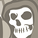# New to Qlik Sense

If you’re new to Qlik Sense, start with this Discussion Board and get up-to-speed quickly.

cancel
Showing results for
Did you mean:Contributor

## Sorting data

Hello.

I have a sorting assignment. Now, I don't really know how the data provided looks like as it is taken from somewhere within a cloud, but I need to sort it by some criteria. I'll make up a toy assignment which mimics my assignment :

Assume I have the following data

Category name               Letters

We                                         A

You                                       C

They                                     A-D

The letters correspond to properties of certain numerical values and the categories should sum up those numerical values if they satisfy the conditions on the letter, that is

We = Number of A

You = Number of C

They = Number of A + Number of C

However, if I write the code in the following way

if([Letter]=A, 'We',

if([Letter]=C,'You',

if([Letter]=A or [Letter]=B or [Letter]=C or [Letter]=D,'We','not assigned')))

once it sorts the value of A into category We, it will not consider it any longer,  resulting in the value of category They  to be zero, that is, it won't show, as the value of C was also sorted before hand and will not be considered any longer.

How can this problem be solved? I thought about implementing a list, but could not find how to do this with this kind of data. Another way I thought about was to just sum the values of We and You  to get They, but also could not find how this can be done. Every help is welcome and needed.

Thanks a ton!